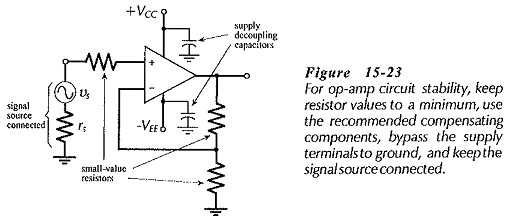## Circuit Stability Precautions:

Power Supply Decoupling – Feedback along supply lines is another source of op-amp circuit instability. This can be minimized by connecting 0.01 μF high-frequency capacitors from each supply terminal to ground (see Fig. 15-23). The capacitors must be connected as close as possible to the IC terminals. Sometimes larger values capacitors are required.### Circuit Stability Precautions:

The following precautions should be observed for circuit Stability:

1. Where low-frequency performance is required, use an internally compensated op-amp. Alternatively, use Miller-effect compensation to give the lowest acceptable cutoff frequency.

2. Use small-value resistors in the feedback network, if possible, instead of using the largest possible resistor values.

3. With an op-amp that must be compensated, use the methods and components recommended by the IC

4. Keep all component leads as short as possible, and take care with component placement. A resistors connected to an op­amp-input terminal should have the resistor body placed close to the input terminal.

5. Use 0.01 μF capacitors (or 0.1 μF capacitors if necessary) to bypass the supply terminals of op-amp (or groups of op-amps) to ground. Connect these capacitors close to the ICs.

6. Always have a signal source connected to a circuit being tested. Alternatively, ground the circuit input. With an open-circuited input, very small stray capacitances can cause instability.

7. Do not connect oscilloscopes or other instrument at the op-amp input terminals. Instrument input capacitance can cause.

8. If a circuit is unstable after all of the above precautions have been observed, reduce the value of all circuit resistors (except compensating resistors). Also, reduce the signal source resistance if possible.

Scroll to Top